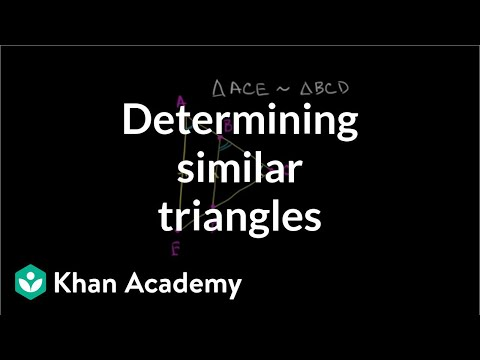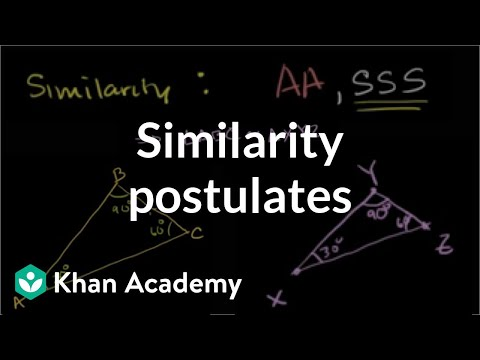## Similarity

Subject: Compulsory Maths

Find Your Query

#### Overview

$\triangle$ABC and $\triangle$XYZ are similar i.e, $\triangle$ABC and $\traingle$XYZ have same shape but the size is different. If any two angles are equal to each other, then they are similar.

### Conditions for similarity of triangles

There are three conditions for similarity of triangles:

i) Angle, Angle similarity testFig:Angle Angle

If two angles of one triangles are respectively equal to two angles of another triangle, then two triangles are similar.

For example:

Here,∠B =∠Y and∠C =∠Z. The remaining angles∠A and∠X are also equal.

∴ $\triangle$ABC∼ $\triangle$XYZ

ii) Side, Side, Side similarity testFig: SSS

If the corresponding sides of two triangles are proportional, then the triangles are similar.

For example:

Here, PQ/XY = QR/YZ = PR/XZ

∴ $\triangle$PQR∼ $\triangle$XYZ

iii) Side, Angle, Side similarity testFig: SAS

If two corresponding sides of two triangles are proportional and the angle contained by these sides are equal, then the triangles are similar.

For example:

Here, XY/AB = YZ/BC and∠Y =∠B

∴ $\triangle$XYZ∼ $\triangle$ABC

#### Similar polygons

Two polygons are similar under following conditions:

i) When two or more polygons are equiangular, they are similar.In the figure, ∠A =∠P,∠B =∠Q,∠C =∠R,∠D =∠S

∴ quad ABCD∼ quad PQRS

ii) When the corresponding sides of two polygons are proportional, they are similar.

In the figure, AB/PQ = BC/QR = CD/RS = DA/SP

∴ quad ABCD∼ quad PQRS

iii) When the corresponding diagonals of the polygons are proportional to their corresponding sies, they are similar.In the figure, AC/PR = BD/QS = AB/PQ

∴ quad ABCD∼ quad PQRS

iv) When the corresponding diagonals divide the polygons into the equal number of similar triangles, the polygons are equal.$\triangle$ABC∼ $\triangle$PQR, $\triangle$ACD∼ $\triangle$PRS, $\triangle$ADE∼ $\triangle$PSV

∴ polygon ABCDE∼ polygon PQRS

Note: Theorem with '*' in similarity chapter do not need proof or experimental verification but the problems related to them are included in the curriculum.

##### Things to remember
• Two geometrical objects are called similar if they both have the same shape, or one has the same shape as the mirror image of the other. More precisely, one can be obtained from the other by uniformly scaling (enlarging or shrinking), possibly with additional translation, rotation and reflection.
• It includes every relationship which established among the people.
• There can be more than one community in a society. Community smaller than society.
• It is a network of social relationships which cannot see or touched.
• common interests and common objectives are not necessary for society.
##### Videos for Similarity##### Similar triangle example problems | Similarity | Geometry | Khan Academy##### Similarity example problems | Similarity | Geometry | Khan Academy##### Questions and Answers
 Statements Reasons 1. In ABE and ACD(i) ABE = ACD(ii) BEA = CDA(iii) ABE ~ ACD 1.(i) Corresponding angles ; BE || CD.(ii) Corresponding angles ; BE || CD.(iii) By A.A similarity 2. $\frac{AC}{AB}$ = $\frac{CD}{BE}$or , $\frac{x + 4}{4}$ = $\frac{3}{2}$or , 2x + 18 = 12oe , 2x = 12 - 8 = 4$\therefore$ $\frac{4}{2}$ = 2 cm. 2. In similar triangles ACD and ABE corresponding sides are in proportion. 3. $\frac{AD}{AE}$ = $\frac{CD}{BE}$or , $\frac{y + 3}{y}$ = $\frac{8.1}{5.4}$or , $\frac{y + 3}{y}$ = $\frac{3}{2}$or , 2y + 6 = 3yor , 6 = 3y - 3y$\therefore$ y = 6 cm. 3. From statement (2).

Here , in OLN and LPM

 1. LNO = LPM 1. From given. 2. OLN = PLM 2. Common Angle. 3. OLN ~ LPM 3. Two equiangular triangles are similar. 4. $\frac{LN}{LP}$= $\frac{LO}{LM}$or , $\frac{LM + MN}{LP}$ = $\frac{LP + PQ}{LM}$or , $\frac{5 + MN}{6}$ = $\frac{6 + 1}{5}$ = $\frac{7}{5}$or , 25 + 5MN = 42or , 5MN = 42 - 25 = 17$\therefore$ MN = $\frac{17}{5}$ = 3.4 cm Corresponding sides of similar triangles are proportional.
 (i) CEA = CBD Given (ii) ACE = BCD Common Angle (iii) CAE = BDC Remaining Angle (iv) AEC~BDC By A.A.A similarity (v) $\frac{CE}{BC}$ =$\frac{CA}{CD}$or ,$\frac{ED + 5}{6}$ =$\frac{3 + 6}{5}$or ,$\frac{ED + 5}{6}$ =$\frac{9}{5}$or , ED + 5 =$\frac{54}{5}$or , ED = 10.8 -5 = 5.8 cm Being AEC ~ BDC

Here , DCO and OAB

 1. CDO = OBA 1. Being DC || AB , DB is transversal and alternate angles. 2/ DCO = OAB 2. Being DC || AB , AC is transversal and alternate angles. 3/ DCO ~ OAB 3. By A.A.A similarity 4.$\frac{AB}{DC}$ =$\frac{BO}{OD}$or ,$\frac{x}{3}$ =$\frac{5}{2}$or , x =$\frac{15}{2}$ = 7.5 x = 7.5 cm 4. Corresponding sides of similar triangle are proportional

Here , p = 7cm , b = 5cm , h= ?
We know that ,
h2 = p2 + b2

or , h2 = 72 + 52 = 49 + 25 = 74
$\therefore$ h = $\sqrt{74}$ = 8.6 cm Ans.

Here , h = 13cm , p = 8cm , b = ?
We know that ,
p2 + b2 = h2
or , 82 + b2 = 132
or , b2 = 132 - 82 = 169 - 64 = 105
b = $\sqrt{105}$ = 10.25 cm Ans.

Here , GH2 + H I2 = GI2
or , GH2 + 242 = 252
or , GH2 = 252 - 242
or , GH2 = 625 - 57
or , GH2 = 625 - 576
or , GH2 = 49
or , GH= $\sqrt{49}$ = 7 cm

In given rectangle ABCD , AB = 12cm , BC= 8cm , diagonal (AC) = ?
In rt. angled triangle ABC
AC2 = AB2 + BC2
or , AC2 = 122 + 82 = 144 + 64
= 208
Diagonal AC = $\sqrt{208}$ = 14.42 cm

In square ABCD , BC = 6cm , AB = 6cm , diagonal(AC) =?
In rt. angled triangle ABC ,
AC2 = Ab2 + BC2
or , AC2 =62 + 62 = 36 + 36 = 72
diagonal AC = $\sqrt{72}$ =$\sqrt{2}$ = 8.48 cm.

In rectangle ABCD , AB = 8cm , diagonal AC = 12cm ,BC = ?
In rt. angled triangle ABC
AB2 + BC2 = AC2
or , 82 + BC2 = 122
or , BC2 = 122 - 82 = 144 - 64 = 80
or , BC = $\sqrt{80}$ = 8.94 cm Ans.

Let us consider AB be the telephone post and CA be wire.
Here CB is the perpendicular distance from the rope fixed at ground C to the post AB.
Here , AB = 7m and AC = 7.6 m
Now , in rt.angled triangle ABC ,
AB2 + BC2= AC2
or , 72 + BC2 = 7.62
or , BC2 = 7.62 - 72 = 57.76 - 49 = 8.76
$\therefore$ BC = $\sqrt{8.76}$ = 2.96 m Ans.

In rectangle ABCD ,
Length (AB)= 5.1 cm , diagonal (AC) = 6.1 cm and breadth (BC) = ?
Here , in rt., angled triangle ABC ,
(5.1)2 + (BC)2 = (6.1)2
or , BC2 = (6.1)2 - (5.1)2
or , BC2 = 37.21 - 26.01 = 11.2
$\therefore$ BC = $\sqrt{11.2}$ = 3.35 cm
Area of rectangle ABCD = length $\times$ breadth = 5.1 $\times$ 3.35 cm 2
= 17.085 cm 2

In DEF , EF =12cm , DE = 9cm , AD = ?
In rt. angled triangle DEF , using pythagoras theorem ,
DF2 = EF2 + DE2
or , DF2 = 122 + 92
or , DF = $\sqrt{144 + 81}$ = $\sqrt{225}$ = 15.
Now , in rt. angled triangle ADF ,
AF = 8cm , DF = 15 cm , DA = ?
Here , in ADF ,
DA2 = DF2 + AF2
or , x2 = 152 + 82
or , x2 = 225 + 64
$\therefore$ x = $\sqrt{289}$ = 17cm.

IN JHI ,HI = 5cm , JI = 2cm , HJ = ?
In rt. angled triangle JHI ,
JH2 = HI2 + JI2
or , JH2 = 52 + 22
or , JH2 = 29
$\therefore$ JH = $\sqrt{29}$ cm.
Again ,
HG2 = JH2 + GJ2
or , HG2 = ($\sqrt{29}$ )2 + 32
or , HG2 = 29 + 9 = 38
or ,HG = $\sqrt{38}$ 6.16
$\therefore$x = 6.16 cm Ans.

Here , AC2 + AB2 = (15)2 + (8)2 = 289
and BC2 = (17)2 = 289
AC2 + BC2 = AB2
h2 = p2 + b2 , so given triangle is right angled triangle in which BAC = 90o

##### Quiz

© 2019-20 Kullabs. All Rights Reserved.